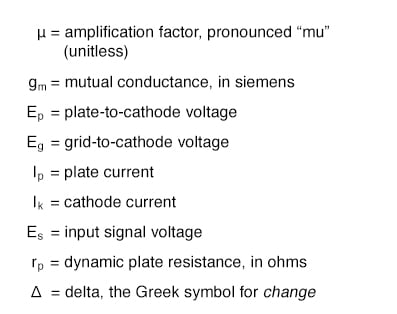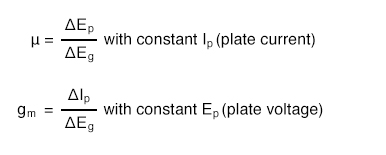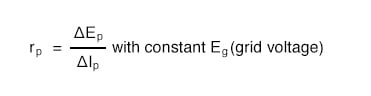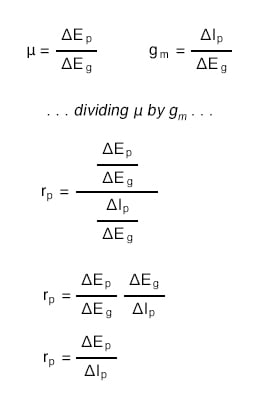Vol. Semiconductors
Chapter 13 Electron Tubes

# Tube Parameters

For bipolar junction transistors, the fundamental measure of amplification is the Beta ratio (β), defined as the ratio of collector current to base current (IC/IB). Other transistor characteristics such as junction resistance, which in some amplifier circuits may impact performance as much as β, are quantified for the benefit of circuit analysis. Electron tubes are no different, their performance characteristics having been explored and quantified long ago by electrical engineers.

Before we can speak meaningfully on these characteristics, we must define several mathematical variables used for expressing common voltage, current, and resistance measurements as well as some of the more complex quantities:The two most basic measures of an amplifying tube’s characteristics are its amplification factor (µ) and its mutual conductance (gm), also known as transconductance. Transconductance is defined here just the same as it is for field-effect transistors, another category of voltage-controlled devices. Here are the two equations defining each of these performance characteristics:Another important, though more abstract, measure of tube performance is its plate resistance. This is the measurement of plate voltage change over plate current change for a constant value of grid voltage. In other words, this is an expression of how much the tube acts like a resistor for any given amount of grid voltage, analogous to the operation of a JFET in its ohmic mode:The astute reader will notice that plate resistance may be determined by dividing the amplification factor by the transconductance:These three performance measures of tubes are subject to change from tube to tube (just as β ratios between two “identical” bipolar transistors are never precisely the same) and between different operating conditions. This variability is due partly to the unavoidable nonlinearities of electron tubes and partly due to how they are defined. Even supposing the existence of a perfectly linear tube, it will be impossible for all three of these measures to be constant over the allowable ranges of operation. Consider a tube that perfectly regulates current at any given amount of grid voltage (like a bipolar transistor with an absolutely constant β): that tube’s plate resistance must vary with plate voltage because plate current will not change even though plate voltage does.

Nevertheless, tubes were (and are) rated by these values at given operating conditions, and may have their characteristic curves published just like transistors.

#### Lessons in Electric Circuits

##### Volumes »
Published under the terms and conditions of the Design Science License